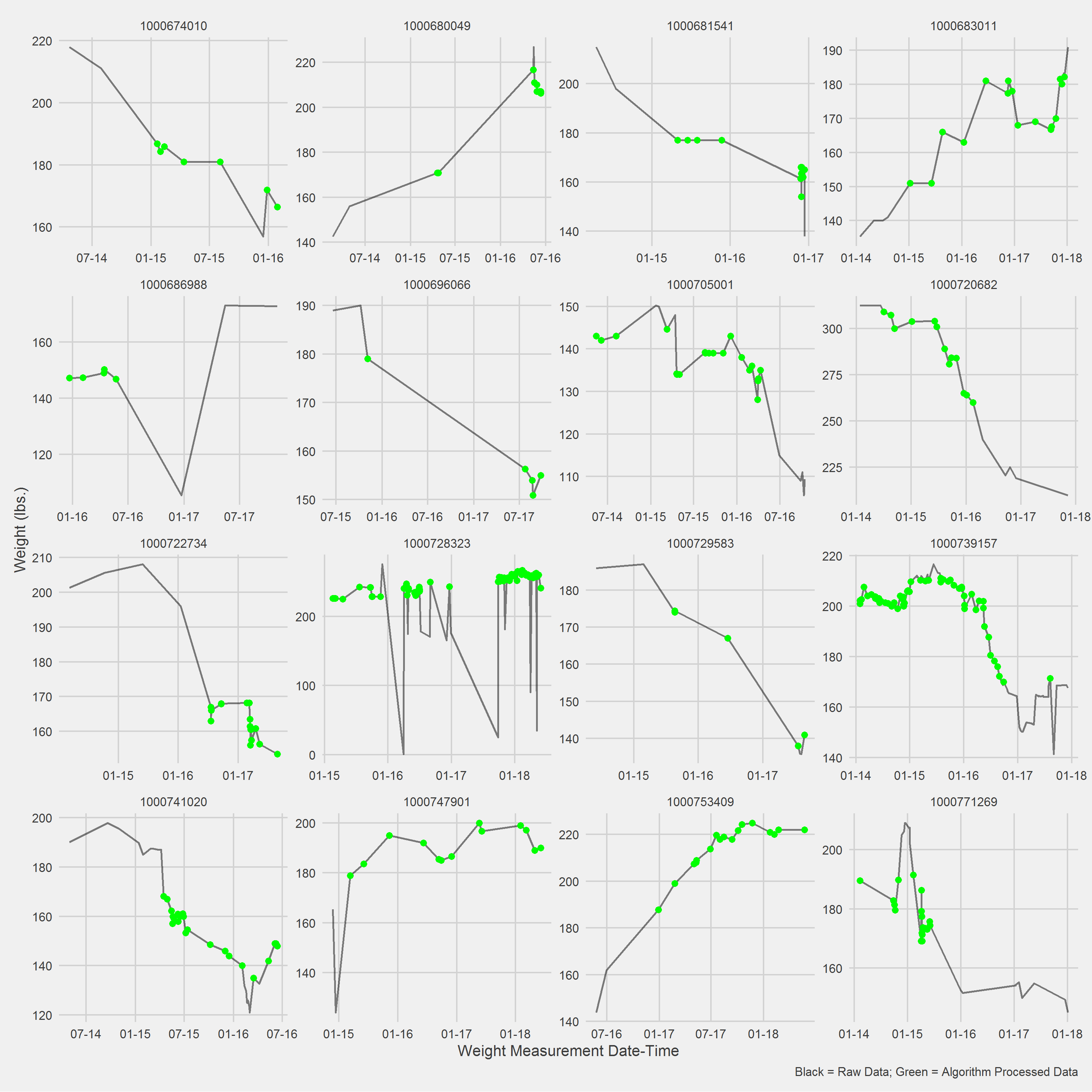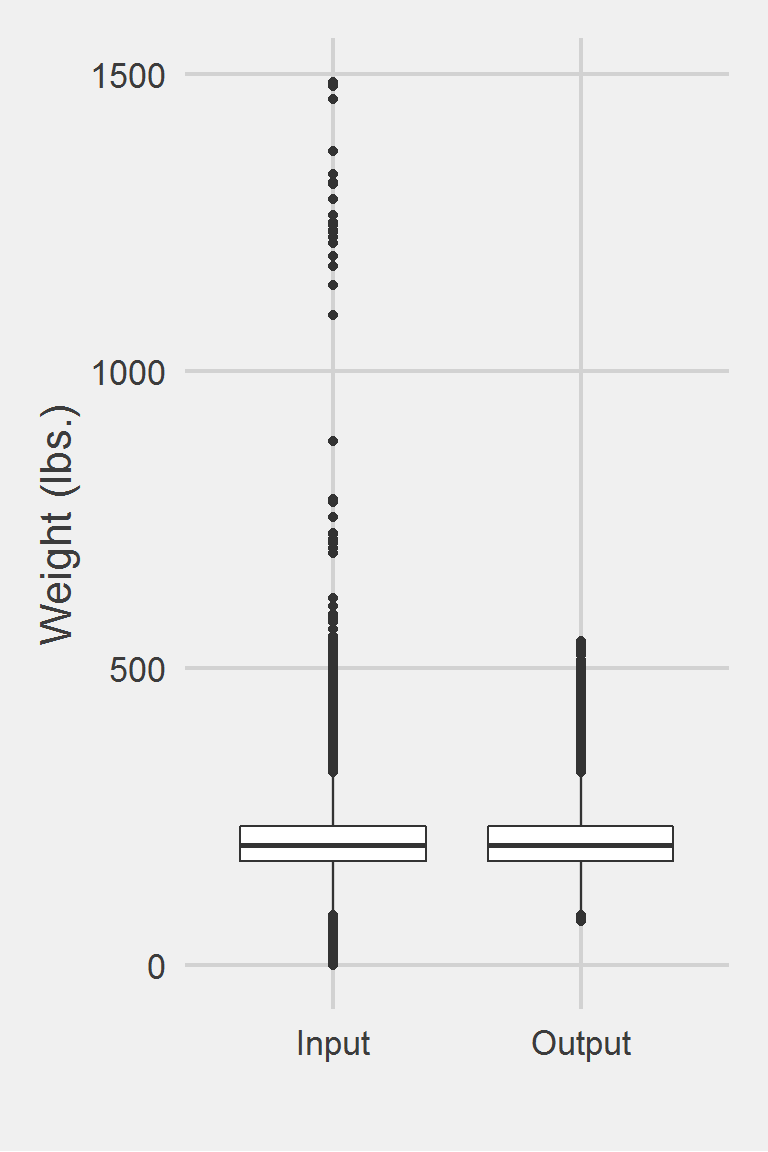## Littman 2012

### Excerpt from published methods

For weight, height, and body mass index, we first removed biologically implausible values (weight <75 lb or >600 lb, height <49 in or >94 in, and body mass index >80 kg/m ). Next, we applied algorithms to identify measures that were plausible but appeared to be erroneous on the basis of a review of all recorded weights and heights during the relevant time period. After reviewing records that had large standard deviations (SDs) (explained in more detail below), we used the algorithm that follows to exclude values that were likely erroneous while keeping values that were plausible. We excluded any weight measurements that met the following 2 criteria: 1) the difference between the mean weight and weight in question was greater than the SD and 2) the SD was greater than 10% of the mean. For example, 1 participant’s weight in pounds was recorded as 300 and 160 lb, both measured on December 7, 2005, 310 lb measured on June 12, 2006, 276 lb measured on August 8, 2006, 291 lb measured on August 15, 2006, and 291 lb measured on September 13, 2007, resulting in mean (SD) of 271.3 (55.7) lb. The weight of 160 lb recorded on December 7, 2005, was considered erroneous and dropped because the difference between the index weight and mean weight was greater than the SD (271 − 160 = 113.3 lb) and the SD was greater than 10% of the mean of all weights ([55.7/271.3] × 100 = 20.5%).

### Translation in Pseudocode

DEFINE person i IN 1:I, j date IN 1:J, weight k IN 1:K (additional recorded weight, same day)
FOR i IN 1:I
FOR j IN 1:J
FOR k IN 1:K
weight_ijk := weight for ith person on jth date and kth weight
END FOR
END FOR
IF (weight_ijk < 75 lbs OR weight_ijk > 600 lbs)
weight_ijk := NA
MEAN_i := MEAN({weight_i}_jk)
SD_i := SD({weight_i}_jk)
IF (ABS(MEAN_i - weight_ijk) > SD_i & SD_i > 0.10 * MEAN_i)
weight_ijk := NA
END FOR

### Algorithm in R Code

This algorithm below assumes all weight data has already been collected, in contrast with the pseudocode.

#' @title Littman 2012 Measurment Cleaning Algorithm
#' @param DF object of class data.frame, containing id and measures
#' @param id string corresponding to the name of the column of patient identifiers in df
#' @param measures string corresponding to the name of the column of measurements in df
#' @param tmeasures string corresponding to the name of the column of measurement collection dates or times in df. If tmeasures is a date object, there may be more than one weight on the same day, if it precise datetime object, there may not be more than one weight on the same day
#' @param outliers object of type list with numeric inputs corresponding to the upper and lower bound for each time entry. Default is list(LB = c(75), UB = c(600))
#' @param SDthreshold numeric scalar to be multiplied by the meanMeasures per id. E.g., from Littman 2012, "...We excluded any weight measurements that met the following 2 criteria: 1) the difference between the mean weight and weight in question was greater than the SD and 2) the SD was greater than 10% of the mean...." implies SDthreshold= 0.10
#' @return input data.frame with additional columns: InputMeasurement, the original weight data; OutputMeasurement, algorithm output; meanWeight, mean(weights) per id; SDWeight, SD(weights) per id; SD_threshold_, Mean(weights) * SDthreshold
Littman2012.f <- function(DF,
id,
measures,
tmeasures,
outliers = list(LB = c(75), UB = c(600)),
SDthreshold = 0.10) {

if (!require(dplyr))      install.packages("dplyr")
if (!require(data.table)) install.packages("data.table")

tryCatch(
if (!is.numeric(DF[[measures]])) {
stop(
print("measure data must be a numeric vector")
)
}
)

tryCatch(
if (!is.list(outliers)) {
stop(
print("outliers must be placed into a list object")
)
}
)

# Compute the mean and sd for each persons weight, if more than 1 value
# if not more than 1 value, mean is finite, sd is undefined
# convert to data.table
DT <- data.table::as.data.table(DF)
setkeyv(DT, id)

# first set outliers to NA
DT[,
measures_aug_ := ifelse(get(measures) < outliers[]
| get(measures) > outliers[],
NA,
get(measures))
]

# calc mean of measures per group
DT[, meanMeasures := mean(measures_aug_, na.rm = TRUE), by = id]
# calc SD of weight per group
DT[, SDMeasures := sd(measures_aug_,   na.rm = TRUE), by = id]
# calc SD threshold
DT[, SD_threshold_ := SDthreshold * meanMeasures, by = id]

# exclude any measurements that meet the following 2 criteria:
# 1) the difference between the meanMeasures and measures_aug_ in
# question is greater than the SDMeasures
# AND
# 2) the SDMeasures was greater than SD_threshold_ of the mean
DT[, cond1 := ifelse(abs(measures_aug_ - meanMeasures) > SDMeasures, T, F)]
DT[, cond2 := ifelse(SDMeasures > SD_threshold_, T, F)]
DT[, measures_aug_ := ifelse((cond1 & cond2), NA, measures_aug_)]

# return augmented measurements
DF <- as.data.frame(DT)
names(DF)[names(DF) == measures] <- "InputMeasurement"
names(DF)[names(DF) == "measures_aug_"] <- "OutputMeasurement"
DF
}

### Algorithm in SAS Code

/*
title: Littman 2012 Weight Measurment Algorithm
param: df: table containing id and weights
param: id: string corresponding to the name of the column of patient IDs in df
param: weights: string corresponding to the name of the column of weights in df
param: tweights: string corresponding to the name of the column of weight dates
or times in df. If tweights is a date object, there may be more than one
weight on the same day, if it precise datetime object, there may not be
more than one weight on the same day.
param: outliers: numeric inputs corresponding to the upper and lower bound
param: SDthreshold: numeric scalar to be multiplied by the meanWeight
per id. E.g., from Littman 2012, "...We excluded any weight
measurements that met the following 2 criteria: 1) the difference
between the mean weight and weight in question was greater than the SD
and 2) the SD was greater than 10% of the mean...." implies
SDthreshold= 0.10
*/

%MACRO Littman2012(df = ,
id = ,
measures = ,
tmeasures = ,
outliers = ,
SDthreshold = );

* Step 1: set outliers to NA;
DATA dt;
SET &df;
IF &measures < %scan(&outliers, 1, ' ')
OR &measures > %scan(&outliers, 2, ' ')
THEN measures_aug_ = .;
ELSE measures_aug_ = &measures;
RUN;

* Step 2: compute mean, sd, SD threshold of measures per group id;
PROC MEANS DATA = dt NOPRINT NWAY;
CLASS &id;
VAR measures_aug_;
OUTPUT OUT = dt2 (DROP = _TYPE_ _FREQ_)
MEAN = meanMeasures
STDDEV = SDMeasures;
RUN;

PROC SQL;
CREATE TABLE descByGroup AS
SELECT a.*,
b.meanMeasures,
b.SDMeasures,
(&SDthreshold * b.meanMeasures) AS SD_threshold_
FROM dt AS a INNER JOIN dt2 AS b ON a.&id = b.&id;
QUIT;

/*
Step 3: exclude any measurements that meet the following 2
criteria: 1) the difference between the meanMeasures
and measures_aug_ in question is greater than the SDMeasures
AND 2) the SDMeasures is greater than the SD_threshold_
*/

DATA outputData;
SET descByGroup;
IF (ABS(measures_aug_ - meanMeasures) > SDMeasures)
THEN cond1 = 1;
ELSE cond1 = 0;
IF (SDMeasures > SD_threshold_)
THEN cond2 = 1;
ELSE cond2 = 0;
IF (cond1 = 1 AND cond2 = 1)
THEN measures_aug_ = .;
RENAME measures_aug_ = outputMeasurement;
RUN;

%MEND Littman2012;

### Example in R

Using a small data frame with the example given in the supplemental materials to Littman 2012:

PatientICN Weight WeightDate
1010528308 300 2005-12-07
1010528308 160 2005-12-07
1010528308 310 2006-06-12
1010528308 276 2006-08-08
1010528308 291 2006-08-15
1010528308 291 2007-09-01

Then applying the algorithm with the default settings

PatientICN InputMeasurement WeightDate OutputMeasurement meanMeasures SDMeasures SD_threshold_ cond1 cond2
1010528308 300 2005-12-07 300 271.33 55.69 27.13 FALSE TRUE
1010528308 160 2005-12-07 NA 271.33 55.69 27.13 TRUE TRUE
1010528308 310 2006-06-12 310 271.33 55.69 27.13 FALSE TRUE
1010528308 276 2006-08-08 276 271.33 55.69 27.13 FALSE TRUE
1010528308 291 2006-08-15 291 271.33 55.69 27.13 FALSE TRUE
1010528308 291 2007-09-01 291 271.33 55.69 27.13 FALSE TRUE

Next example will run on the sample

Littman2012.df <- Littman2012.f(DF,
id = "PatientICN",
measures = "Weight",
tmeasures = "WeightDateTime")

Displaying a Vignette of 16 selected patients with at least 1 weight observation removed.Distribution of raw weight data versus algorithm processed data


Descriptive statistics by group
group: Input
vars       n   mean   sd median trimmed   mad min    max  range skew
X1    1 1175995 207.82 48.6  202.3  204.62 44.18   0 1486.2 1486.2 0.98
kurtosis   se
X1      5.6 0.04
------------------------------------------------------------
group: Output
vars       n   mean    sd median trimmed   mad  min max range skew kurtosis
X1    1 1161661 207.97 48.14  202.6  204.77 44.03 75.2 546 470.8 0.81     1.41
se
X1 0.04

Left boxplot is raw data from the sample of 2016, PCP visit subjects while the right boxplot describes the output from running Littman2012.f()